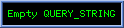# Kim Maltman's Mini-Course on Trig Basics

This mini-course on the basics of trigonometry aims to provide students with an easy-to-grasp, intuitive understanding of

• the meaning of angles and angular measure (a topic/concept crucial to the rest of the course);
• the fundamental geometrical meaning of the six trigonometric (trig) functions (the sine, cosine, secant, cosecant, tangent and cotangent);
• the key algebraic relations amongst these functions; and
• a number of important, and commonly used, properties of these functions based on their geometrical meanings and algebraic interrelations, including (i) how to use elementary geometry, in combination with the basic geometrical definitions of the trig functions, to work out exact values for these functions for certain special angles; (ii) how each of the six trig functions changes as the angle θ on which it depends changes; (iii) the geometrical origins of a number of important "trig identities", and how one goes about actually using and understanding the meaning of these identities.

A basic outline of the course is given in the Table of Contents. For more on how the material in the course proper is organized, see the comments following the Table of Contents.

TABLE OF CONTENTS

Chapter 1: The Basics of Angles and Angular Measure

• (a) Motivation for the use of angles and angular measure to specify directions in the plane
• (b) Basic conventions for assigning angles (numbers) to directions in the plane
• (c) Degrees and radians as units of angular measure, and how to convert from one to the other
• (d) Why there is more than one angle corresponding to a given direction in the plane

Chapter 2: Key Geometrical Background and the Fundamental Geometrical Meaning of the Six Trig Functions

• (a) Demonstration of the fact that the sum of the interior angles of any triangle is equal to π radians (180o)
• (b) Pythagoras' Theorem (including a simple geometric proof)
• (c) The basic geometric definitions of the six trigonometric functions as ratios of sides of right-angle triangles
• (d) Exact values of the trig functions of the angles π/6, π/4 and π/3 radians from elementary geometry

Chapter 3: Generalizing the Trig Functions to Non-First-Quadrant Directions

• (a) Algebraic interrelations amongst the six trig functions
• (b) The unit circle picture of the geometry of the sine and cosine functions
• (c) Using the unit circle picture to generalize the definitions of the trig functions to angles outside of those first quadrant angles which naturally occur as the interior angles of right angle triangles
• (d) An example of the utility of the generalized unit circle definitions: trig functions values for directions along the +x, -x, +y and -y axes
• (e) An alternate triangle-based perspective for the generalization of the trig functions to non-first quadrant angles
• (f) Exact values from elementary geometry for the trig functions of angles corresponding to directions one-third, one-half or two-thirds of the way through the second, third and fourth quadrants

Chapter 4: Range, Periodicity, and How the Trig Functions Change With Angle

• (a) The behavior of the six trig functions for angles corresponding to directions in between the special directions for which exact values were already worked out above using elementary geometry
• (b) The "ranges" (sets of all possible allowed values) of the six trig functions
• (c) The "periodic" (regular repeating) properties of the six trig functions

Chapter 5: Trig Identities and Their Implications

• (a) Background discussion on the idea of an "identity", and how it differs from the more general idea of an "equation"
• (b) Three important identities which follow, via elementary geometry, from Pythagoras' Theorem
• (c) A preliminary summary of the identities to be discussed in Sections (d), (e) and (f) of this chapter, including some basic discussion and illustrations of how these identities are meant to be interpreted and used
• (d) Identities between trig functions of pairs of angles associated with different, but closely related, right-angle triangles
• (e) The sine and cosine additional formulas
• (f) Other identities easily worked out from the sine and cosine addition formulas, e.g., the tangent and cotangent addition formulas, the double-angle formulas, the half-angle formulas, ...

ORGANIZATION OF THE MATERIAL IN THE COURSE

The course consists of five chapters, each containing a number of sections. Each such section focusses on a key topic or small set of related topics. The material is organized as follows:

• The links above for each chapter take you to a "chapter summary page". The chapter summary pages contain:
• a short overview of what is to be covered in the chapter,
• links to earlier sections of the course which are of particular relevance as background to the material in the current chapter,
• links to take you to the materials in the various sections of the chapter in question, and
• a link to the PDF file containing answers to the exercises from all sections of the current chapter. (The set of answers to the exercises in a given chapter form the last section of that chapter.)
• In most cases, the link to a particular section of a given chapter (found both in the Table of Contents above, and on the summary page for the chapter as a whole) will take you to another summary page specific to that section of the chapter. This "chapter section summary page" will contain:
• a short overview of what is to be covered in the section,
• links to earlier sections of the course which are of particular relevance as background to the material in the current section,
• a link to the PDF file containing the actual material in the section, and
• another link to the PDF file containing the answers to the exercises from all sections of the current chapter.